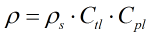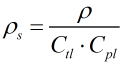# C012 – API/Table 54 Density Referral

## Description

This converts density values between standard conditions (1.01325 bar and 15°C) and other observed or line conditions.

Kelton calculation reference C012

FLOCALC calculation reference F028

KIMS calculation reference K211

### Ctl The correction for the pressure of the liquid is calculated using

(see C219 for calculation details)

“Report on the Development, Construction, Calculation and Preparation of the ASTM-IP Petroleum Measurement Tables (ASTM D 1250; IP 200)”: First edition 1960, Reprinted 1962 and 1968.

### Cpl The correction for the temperature of the liquid is calculated using

(see C220 for calculation details)

Measurement of Petroleum Measurement Standards Chapter 11.2.1 – Compressibility Factors for Hydrocarbons: 0-90° API Gravity Range (1984)

The correction for pressure is calculated using the Measurement of Petroleum Measurement Standards Chapter 11.2.1M – Compressibility Factors for Hydrocarbons: 638-1074 Kilograms per Cubic Metre Range (First Edition August 1984)

Measurement of Petroleum Measurement Standards Chapter 11.2.2 – Compressibility Factors for Hydrocarbons: 0.350-0.637 Relative Density (60°F/60°F) and -50°F to 140°F Metering Temperature (First Edition October 1986)

Measurement of Petroleum Measurement Standards Chapter 11.2.2M – Compressibility Factors for Hydrocarbons: 350-637 Kilograms per Cubic Metre Density (15°C) and -46°C to 60°C Metering Temperature (First Edition October 1986)

## Options

### Solve for

• Standard density

Calculate the standard density from the density at a given pressure and temperature

• Density

Calculate the density at a given pressure and temperature from the standard density

### Precision

• Full

This enables you to bypass the rounding methods outlined in the algorithms and use the full precision during the calculation

• Rounding

Select this option to follow the algorithms to the in the standard

### Density Range

• Auto

Automatically switch between the correct calculation for the range

• 350 to 637 kg/m³

Force the low density range to be used for the calculation.

• 638 to 1074 kg/m³

Force the high density range to be used for the calculation

### Vapour Pressure

• Include

This option allows the entry of the vapour equilibrium pressure, If this is not selected the value of 1 standard atmosphere (101325 Pa) is assumed.

## Calculations

### Density

When solving for density the temperature correction according to Table 54 is applied followed by the pressure correction according to API MPMS Chapter 11.2.1.### Standard Density

When solving for standard density an iterative calculation is performed making use of the Table 54 and API MPMS Chapter 11.2.1 algorithms. An initial estimate is taken for standard density based upon the entered density. This value is then fed into the Table 54 temperature correction algorithm to gain the Ctl. The estimate for standard density is then taken into the API 11.2.1 pressure correction algorithm to gain the Cpl. The Ctl and Cpl are then fed into the below formula to gain a new estimate of standard density. The process is repeated until standard density values converge to within 10-6.Back